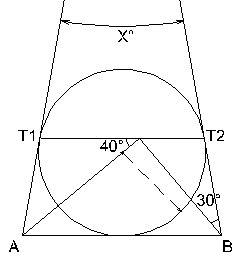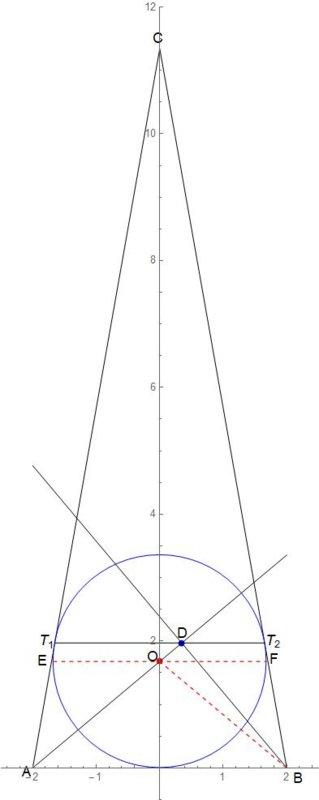# Circle inscribed in a triangle exercise

• Richard Quispe

## Homework Statement

In the drawing you can see a circumference inscribed in the triangle ABC (See the picture in the following link). Calculate the value of X

https://goo.gl/photos/CAacV2dJbUrywfXv9

2. The attempt at a solution

It seems I found a solution for this exercise with the help of a CAD software. However, according to the software T1T2 // AB, <T1AB = <T2BA and X=20°. But I really don't understand why.

I hope you can give me some insights on how to solve this exercise.

Richard

## Homework Statement

In the drawing you can see a circumference inscribed in the triangle ABC (See the picture in the following link). Calculate the value of X2. The attempt at a solution

It seems I found a solution for this exercise with the help of a CAD software. However, according to the software T1T2 // AB, <T1AB = <T2BA and X=20°. But I really don't understand why.

I hope you can give me some insights on how to solve this exercise.
The figure inscribed in the triangle is a circle. A circle has a circumference.

Above, you say that T1T2 || AB, and that the two base angles of the triangle are congruent. What else is given in the problem? The picture shows a couple of angles of 40° and 30°. Is this also given information?

What's the purpose of the circle? It doesn't look like it has anything to do with this problem. Line segment T1T2 doesn't appear to go through the center of the circle, and the upper vertex of the smaller triangle doesn't appear to have anything to do with the circle.

Thanks for the reply, the only given data is what is shown in the picture. Yes T1T2 doesn't go through the center, but the segment that comes from A do.

The additional information I mentioned comes from my attempted solution (CAD software) but I'm not sure

How was this problem presented to you? Is it from a book? I can't help thinking that there is information missing.

Where is the center of the circle? Is it where the the dashed line meets the line coming from A?

Yes, it is an exercise from a book, the center is at the beginning of the dashed line. Can I safely say this exercise has missing information?

The part where the angles have equal measure is the assumption that this triangle ABC is isosceles.
If T1T2 is parallel to AB, then you have all kinds of tools to fill in the measures of the angles.

I suppose these assumptions are easy enough to justify based on the definition of the inscribed circle. I think it would be nicer if it were explicit in the problem.

From there, you can use the right angle from the triangle (let's call it ABD) to find the measure of the angle T2DB...this is clearly congruent to the angle DBA.
Now you fill in your angles to find the base angles of the isosceles triangle. Your angle x will be what's left to make 180.

From there, you can use the right angle from the triangle (let's call it ABD)
It's not given that ∠ABD is a right angle, where I assume that you mean that D is the point on segment T1T2. How can we make this assumption?

Last edited:
The part where the angles have equal measure is the assumption that this triangle ABC is isosceles.
If T1T2 is parallel to AB, then you have all kinds of tools to fill in the measures of the angles.

I suppose these assumptions are easy enough to justify based on the definition of the inscribed circle. I think it would be nicer if it were explicit in the problem.

If you are thinking T1T2 is parallel to AB because T1 and T2 are where the incircle intersects the sides of the triangle, I don't suppose that is "easy enough to justify". It is false in general and seems likely to be equivalent to the angles at A and B being equal. And that isn't given either.

Hi,

I think I finally understood the solution for this exercise. I've uploaded an additional image with the solution

https://goo.gl/photos/CAacV2dJbUrywfXv9

First find out <OBA=50°, since OB bisects <ABT2, <OBD=10°.
The trickiest part of this exercise was finding out that the quadrilateral ODT2B can be inscribed in a circle. Which can be proved because <ODT2+<OBT2=180°. Then Φ=10°, <T1T2C=<T2T1C=80°. Therefore X=20°.
Additionally <T2DB=50° which demonstrates T1T2 // AB.

Thank you all for the effort put in this exercise.

Richard

Hi,

I think I finally understood the solution for this exercise. I've uploaded an additional image with the solution

https://goo.gl/photos/CAacV2dJbUrywfXv9

First find out <OBA=50°.

Apparently you mean <DBA = 50°, not <OBA=50°. And how do you see that?

LCKurtz, you are right <OBA=50°, we can obtain that value by checking the angles in the triangle ADB

LCKurtz, you are right <DBA=50°, we can obtain that value by checking the angles in the triangle ADB

First find out <OBA=50°, since OB bisects <ABT2, <OBD=10°.

Can you or perhaps someone else explain to me how to determine ##OB## bisects ##\angle ABT_2## please?